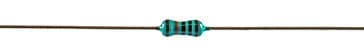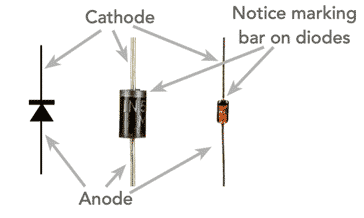# Ohmic & Non-Ohmic Conductors

### Not all conductors and electronic components follow the ideal Ohms law characteristic of a linear relationship between voltage and current. There are many examples of Ohmic conductors and non-Ohmic ones.

Resistance Tutorial Includes:
What is resistance     Ohms Law     Ohmic & Non-Ohmic conductors     Resistance of filament lamp     Resistivity     Resistivity table for common materials     Resistance temperature coefficient     Electrical conductivity     Series & parallel resistors     Parallel resistors table

Ohms Law states that there is a linear relationship between voltage and current. Conductors and electronic components that follow this law are called Ohmic conductors, whereas others are called non-Ohmic conductors

The Ohmic conductors may also be called linear electronic components, whereas the others are non-linear as they do not have a linear relationship between voltage and current.

In understanding the basics of electronics as well as many elements of electrical science it is necessary to understand why some electronic components and conductors are Ohmic following Ohms law and others are non-Ohmic having a non-linear relationship between voltage and current.

## Main examples of Ohmic and non-Ohmic conductors

There are very many examples of both Ohmic and non-Ohmic conductors. Both types are widely used in all forms of electrical and electronic components and systems.

Check out our video on Ohmic & Non-Ohmic Conductors

In view of the importance to electrical design as well as electronic circuit design, it is important to understand which conductors as well as which electronic components fall into which categories.

#### Ohmic conductors & electronic components

Looking at Ohmic conductors it is worth giving a more precise definition of what they are:

### Ohmic conductors definition:

Ohmic conductors are those electrical conductors that follow Ohms law. In other words there is a linear relationship between voltage and current for all values

In view of the Ohmic conductors definition it means that if the voltage across the conductor, electronic component, etc is doubled, then the current will also double.

Very broadly speaking items made from metals, carbon, and many metal alloys fall into the Ohmic conductors category.

The relationship between the voltage current and resistance follows Ohms law - it is linear and can be calculated using Ohms Law.

$V=IR$
Where:
V = voltage expressed in Volts
I = current expressed in Amps
R = resistance expressed in Ohms

If the voltage and current for an Ohmic electrical or electronic component is plotted, then a straight line is seen on the graph. A low resistance component will have a much shallower line and a higher resistance device will have a much steeper line on the Ohmic conductor graph.Plot of voltage and current for a linear resistance This is effectively an Ohmic conductor graph

Some of the main examples of ohmic conductors and electronic components include:

• Connecting wire:   The connecting wire normally made of copper used for making connections between different electrical and electronic components, etc is an ohmic conductor exhibiting a linear relationship between the voltage across it and the current flowing through it. Under normal operating conditions, the heat dissipated is low and it remains at a constant temperature.

Although the voltage drop along a section of connecting wire will be low, there is nevertheless some resistance and even though the levels of resistance are much lower than for other items, the connecting wire, such as copper wire will still follow Ohm's law.

• Resistors :   Basic resistors are one of the main electronic components used in electronic circuit designs. In view of the way in which the electronic circuits work, they need to be ohmic and have a linear relationship between voltage and current to ensure the correct functioning of the circuit.Leaded metal film resistor which is an Ohmic electronic component

There are many forms of resistor, most of which are ohmic electronic components. They are used within electronic circuit designs to provide a fixed level of resistance within a circuit to set voltages, limit current and the like. There are some forms of resistor that are non-ohmic that are used for some specialised applications.

#### Note on Resistors & Resistor Types:

Resistors are used in electrical and electronic circuits for a variety of purposes, but in each case they resist the current flow. There are many different types of resistor - their parameters mean that some types are more suitable for particular applications than others.

Read more about Resistors & Resistor Types

These are but a few examples of Ohmic conductors and devices. Ohmic conductors and electronic components are the most straightforward of items. They simply obey Ohm's law and operate in a linear fashion where V = I ⋅ R.

#### Non-Ohmic conductors and electronic components

There is a surprising number of electrical and electronic components that are non-ohmic. Essentially they exhibit a value of resistance that varies with the voltage applied.

Again it is worth defining what a non-Ohmic conductor or electronic component is:

### Non-Ohmic conductors definition:

Non-Ohmic conductors are those electrical conductors that do not follow Ohms law. In other words the relationship between voltage and current is not linear for all values

In other words doubling the voltage will not result in a doubling of the current. This can occur for a variety of reasons dependent upon the actual conductor or component in question.

Some of the main examples of non-ohmic conductors and electronic components include:

• Incandescent light bulb:   The incandescent light bulb is a prime example of a non-ohmic conductor response. Although filament lamps, which are also called incandescent lamps, are not widely used these days because they are very inefficient in terms of their conversion of electrical energy to light energy, they are a good example of a non-Ohmic conductor or electrical component.

The reason for the non-Ohmic characteristic results from the heat generated by the filament of the lamp.

For normal operation a lamp will be powered by a battery or from the mains power lines. These provide an almost constant voltage and it can be assumed this remains the same all of the time. Initially the lamp has a low resistance, and when the power is applied there is an in-rush of current because of this. This means that the filament becomes very hot - it is heated to white heat, and this is why it emits light. However the resistance also rises so that the current reduces and the lamp settles to normal operation.IV characteristic of a incandescent filament lamp

If the V/I characteristic was to be measured and plotted for various voltages, then it would be found that for low voltages the resistance would be low and the current would be high for the voltage applied. As the potential difference across a filament lamp is increased, the current increases and the energy dissipated, as heat, increases, resulting in a filament operating at a higher temperature. As the temperature increases, resistance of the filament also increases.

Read more about . . . . Why a Filament Lamp is Non-Ohmic.

• Semiconductor diode:   The semiconductor diode is one of the most obvious non-Ohmic devices that is available.

The basic diode consists of a junction between P-type and N-type material, and the basic action is that only allows current through in one direction. The ideal diode would have no resistance in the forward direction and infinite resistance in the reverse direction. This alone would make it a non-Ohmic conductor, but in reality the situation is more complicated.Diode circuit symbol and physical diode orientation

In the forward direction, as the potential difference across the device is increased from zero initially little current flows because the current carriers in the PN junction need to have sufficient energy to traverse the junction. As the potential, more current flows as more electrons have sufficient energy, but the current voltage relationship is far from Ohmic.

In the reverse direction, as the potential difference is increased across the diode very little current flows across the junction, although it does increase slowly. However a point is reached where breakdown occurs and current flows.

The overall characteristic of a PN junction diode is far from Ohmic as seen in the characteristic below.PN diode IV characteristic

#### Note on the PN Junction Diode:

The PN junction diode is the most basic form of semiconductor device. Consisting of a single junction between P-type and N-type material it is able to act as a rectifier allowing current to pass in only one direction.

Read more about PN Junction Diode.

• Most semiconductor devices:   The semiconductor diode is a specific example of a semiconductor device that is often encountered within electronic circuits. However it is not the only example of a non-Ohmic conductor made from semiconductor material. Most other semiconductor device are good examples of non-Ohmic responses. It is not possible to detail each type, but it is probably sufficient to mention that they are non-Ohmic in many if not most of the aspects of their operation.

These are just three examples of non-Ohmic conductors and components. There are various other types which can be encountered. Varistors are but one example. These are used for protecting against mains power or line transients. Normally they have a high resistance, but when a certain voltage is exceeded, the resistance falls so that they absorb the transient and protect the units being powered.

Ohmic and non-Ohmic conductors as well as electrical and electronic components are found in all areas of electrical and electronic science. Both types of conductor are used, and their individual properties are put to specific uses to provide the wealth of capabilities that are needed to support modern day electrical and electronic circuit design, components, and systems. It is surprising what a variety of ways in which these different characteristics can be used.

More Basic Electronics Concepts:
Voltage     Current     Power     Resistance     Capacitance     Inductance     Transformers     Decibel, dB     Kirchoff's Laws     Q, quality factor     RF noise
Return to Basic Electronics Concepts menu . . .